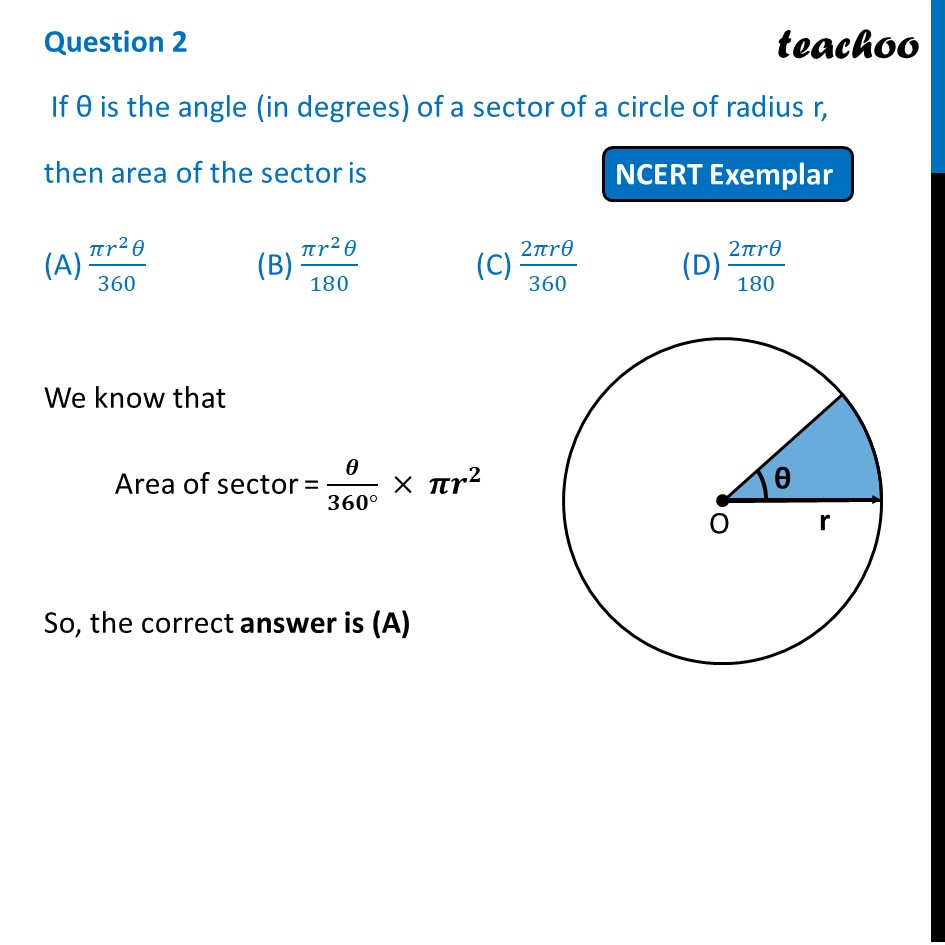## D) 2πrθ/1801. Chapter 12 Class 10 Areas related to Circles (Term 1)
2. Serial order wise
3. NCERT Exemplar - MCQ

Transcript

Question 2 If θ is the angle (in degrees) of a sector of a circle of radius r, then area of the sector is (A) (𝜋𝑟^2 𝜃)/360 (B) (𝜋𝑟^2 𝜃)/180 (C) 2𝜋𝑟𝜃/360 (D) 2𝜋𝑟𝜃/180 We know that Area of sector = 𝜽/(𝟑𝟔𝟎°) × 𝝅𝒓^𝟐 So, the correct answer is (A)